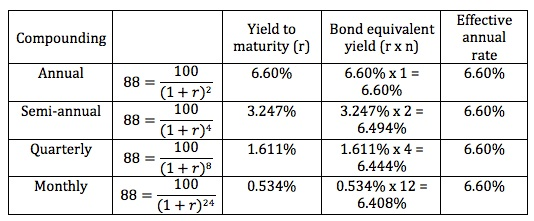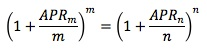Fixed Income I

Reading 44. Introduction to Fixed-Income Valuation

Learning Outcome Statements

f. calculate annual yield on a bond for varying compounding periods in a year;

CFA Curriculum, 2020, Volume 5

### Why should I choose AnalystNotes?

AnalystNotes specializes in helping candidates pass. Period.

### Subject 5. Yield Measures for Fixed-Rate Bonds

Yield measures are used to evaluate the rate of return on bonds.

• They are typically annualized.
• Money market rates are simple interest rates and non-money market rates are compounded.

The periodicity of an annual interest rate is the number of periods in the year.

Consider a two-year, zero-coupon bond priced now at 88 per 100 of par value.Note:

• The effective annual rate is the same.
• The bond equivalent yield and the periodicity are inversely related.
• When comparing different bonds, it is essential to compare the yields for the same periodicity to make a statement about relative value.

To convert an annual yield from one periodicity to another:Example

• A Eurobond pays coupons annually. It has an annual-pay YTM of 8%.
• A U.S. corporate bond pays coupons semi-annually. It has a bond equivalent YTM of 7.8%.
• Which bond is more attractive, if all else equal?

Solution 1

• Convert the U.S. corporate bond's bond equivalent yield to an annual-pay yield.
• Annual-pay yield = [1 + 0.078/2]2 - 1 = 7.95% < 8%
• Therefore, the Eurobond is more attractive since it offers a higher annual-pay yield.

Solution 2

• Convert the Eurobond's annual-pay yield to a bond equivalent yield (BEY).
• BEY = 2 x [(1 + 0.08)0.5 - 1] = 7.85% > 7.8%
• Therefore, the Eurobond is more attractive since it offers a higher bond equivalent yield.

Street convention yields assume that payments are made on scheduled dates, excluding weekends and holidays. The true yield is calculated using a calendar including weekends and holidays. The government equivalent yield is based on actual/actual day count.

The current yield relates the annual dollar coupon interest to the market price. For example, the current yield for a 5%, two-year bond with a price of \$978 is 5.11% ((\$1000 x 5%) / \$978)). This is the simplest of all yield measures, and fails to recognize any capital gain or loss, reinvestment income or accrued interest.

The simple yield is similar to the current yield but includes the straight-line amortization of the discount or premium.

The standard YTM measure assumes that the bond will be held to maturity. It is not an appropriate yield measure for callable bonds, because they may be retired before maturity. For callable bonds a yield to first call, which assumes that the bond will be called on the first call date, is computed.

Callable bonds typically have multiple call dates, each with its own call price. The yield to worst is the lowest potential yield that can be received on a bond without the issuer actually defaulting. It illustrates the worst possible yield an investor may realize. The option-adjusted-yield is the yield-to-maturity after adding the theoretical value of the call option to the price.

This is reasonably straight forward but be careful with the conversion process.

###### ramtor

use the iconv function of BAII plus

###### JimM

Using the ICONV function of BAII plus (it's on the "2" key), remember to set C/Y = 2, not 365.

NOM = BEY
EFF = Annual-pay yield

Set 1 of those, CPT the other.

###### jpducros

Remember that you'll always have :
MMY<BEY<EAY
MMY : Money Market Yield : no compounding - 360 d/year
BEY : Bond Equiv. Yield : Semi-Annual Compounding - 365 d/y
EAY : Effective annual Yield : compounding for the entire year, based on 365 d/y

###### anaraguin

Thank you so so much JimM! :)

###### moneyguy

That still doesn't tell me how to actually apply the iconv button to calculate this stuff, JimM

thanks jim

Thank you JimM

###### davidbenke

@moneyguy
US corporate bond example:
[2nd]
NOM = [.]
C/Y = 
EFF = [CPT]

EFF should equal 7.952

###### philerup

TIL how to use ICONV. Thanks Jim!

###### phill

why do them all have the same EAR and how is that calculated?